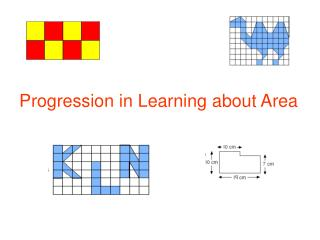DownloadDownload PresentationProgression in Learning about Area

# Progression in Learning about Area

Download Presentation## Progression in Learning about Area

- - - - - - - - - - - - - - - - - - - - - - - - - - - E N D - - - - - - - - - - - - - - - - - - - - - - - - - - -
##### Presentation Transcript

1. Progression in Learning about Area

2. Stage 1: Pre-measurement Experiences Young children need to gain early experiences of measuring area through play, and as a natural part of everyday life. We can fit 9 pictures on the art display board! Our Artwork 15 children can all sit on the rug in the home corner at the same time!

3. Stage 2: Covering Surfaces using Arbitrary Units At this stage, children are asked to cover surfaces using arbitrary units i.e. units which are not identical in terms of their area, for example buttons or leaves. We are going to find out how many leaves it will take to completely cover the table!

4. Stage 3: Covering surfaces using Non-Standard Units (NNS Year 4) At this stage, children progress to covering surfaces with identical sized non-standard units, for example bottle-tops, pennies, playing cards etc. We have covered the home carpet with the footprints we made by drawing around Rosie’s shoe!

5. Stage 4: Deciding upon a Standard Unit for Area (NNS Year 4) By drawing on the ideas met in stage 3, children begin to realise that it is better if we use shapes which will fit together without gaps if we want to count how many shapes are needed to fully cover a shape. This leads onto to introduction of the idea of a square centimetre. I’ve explored the shapes which will fit together without any gaps. So far, I’ve found out that squares, rectangles and triangles will fit together without any gaps. I think there may be more shapes!

6. Stage 5: Measuring Area by Counting Squares (NNS Year 4) Once the idea of the square centimetre has been established as the standard unit for measuring area, children can be asked to start making indirect comparisons between the area of two or more shapes by counting how many centimetre squares will fit within the boundary of each shape. Both these shapes have the same area. They are 8 square centimetres!

7. Stage 6: Extending the Counting Squares Method (NNS Year 5) The counting squares method ‘breaks down’ when we have shapes that cannot be neatly divided into centimetre or half-centimetre squares. In such cases, tracing or drawing around the boundary of the area onto squared paper or overlaying an acetate sheet marked with a centimetre squared grid is probably the best approach. I shall count all the whole squares first. Next, I will count as 1 square any squares which are at least a half covered, like A. I shall ignore all the squares that are less than half covered, like B!

8. Stage 7: Calculating the Area of a Rectangle (NNS Year 5) The first area formula children should encounter is that for a rectangle. It is best to help children to derive this formula for themselves by using 1 cm squared paper and drawing a series of rows for a given number of squares. I have 1 row of 5 squares, that’s 5, and another row of 5 squares, that’s 10 and another row of 5 squares, that’s 15 squares altogether. Hey! I’ve just realised that the total number of squares is 3 x 5 which is the same as the rows times the columns!

9. Stage 8: Introducing Further Units for Area (NNS Year 5) In Year 5, children should be introduced to other units for area, namely mm2 and m2. They should also be expected to develop an appreciation of the approximate size of these units so they can learn to estimate these units and recognise which unit is most suitable for measuring a given area. I reckon the area of the football pitch will be best calculated in square metres! I have calculated the area of this foreign stamp in mm2.

10. Stage 9: Compound Areas Composed of Rectangles (NNS Year 6) Once children can confidently use the area of a rectangle formula, they can be taught to apply this to non-rectangular areas which can be subdivided into rectangles. First I divided the shape up into three large rectangles and two small rectangles. Then I worked out the area of the shape by working out the total area of the three large rectangles and taking away the area of the two small rectangles.

11. Stage 10: Surface Areas of Cuboids (NNS Year 6) The surface area of a 3D shape can be defined as the sum of the areas of its faces. Since the faces of cuboids are rectangles, the area of a rectangle formula can be applied to each face and the resulting areas added to find the total surface area. Children can be encouraged to derive a ‘formula’ for the surface area of a cuboid. I have two faces the same area as A, two faces the same area as B and two faces the same area as C. Um… That’s 2 x A + 2 x B + 2 x C!